# How To Rank Data In Google Sheets With The RANK Function

Google Sheets has three functions to rank data: the RANK function, the RANK.EQ function, and the RANK.AVG function.

All three functions return the rank (position) of a value in a dataset.

RANK and RANK.EQ are equivalent to each other and return the top rank for values that are tied. RANK.EQ is the more modern notation, to explicitly differentiate itself from RANK.AVG.

The RANK.AVG function differs by returning the average rank of any entries that are tied.

Consider this dataset showing the three RANK functions in action, with a tie highlighted in yellow: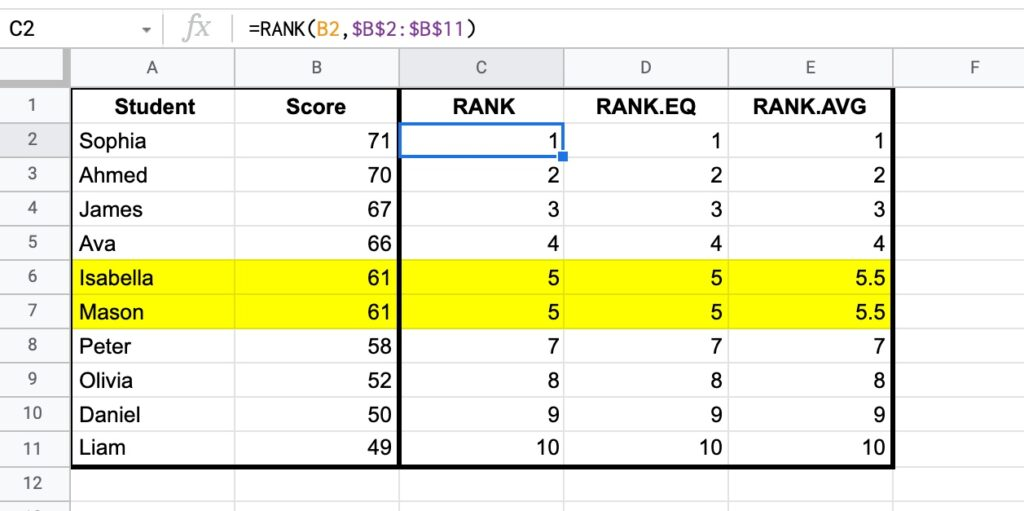Both RANK and RANK.EQ display the tied values with the rank 5, whereas RANK.AVG shows the average rank of 5.5 (i.e. the average of position 5 and position 6).

The RANK formula in column C:

`=RANK(B2,\$B\$2:\$B\$11)`

And RANK.EQ formula in column D, giving the same answer:

`=RANK.EQ(B2,\$B\$2:\$B\$11)`

Finally, RANK.AVG formula is in column E:

`=RANK.AVG(B2,\$B\$2:\$B\$11)`

🔗 Get this example and others in the template at the bottom of this article.

## RANK Functions Syntax

The three RANK functions share the same syntax construction, although note the different function names:

`=RANK(value, data, [is_ascending])`
`=RANK.EQ(value, data, [is_ascending])`
`=RANK.AVG(value, data, [is_ascending])`

The three functions take two mandatory arguments and a third optional argument:

`value`

The value to be ranked.

`data`

An array or range containing the data used for ranking.

`[is_ascending]`

This optional argument determines whether to rank the values descending or ascending, i.e. whether the largest value is first or last.

Use 0 or FALSE to set the largest value as the number 1 rank.

Use 1 or TRUE to set the largest value in the last position, and the smallest value in the number 1 rank.

If this third argument is omitted, the default behavior is descending, i.e. equivalent to 0 or FALSE where the largest value is the number 1 rank.

### RANK Formula Notes

The value to be ranked must be present within the data range or array, otherwise the formula returns a #N/A error.

In the following example, the value 63 cannot be ranked because it doesn’t occur in the range of scores: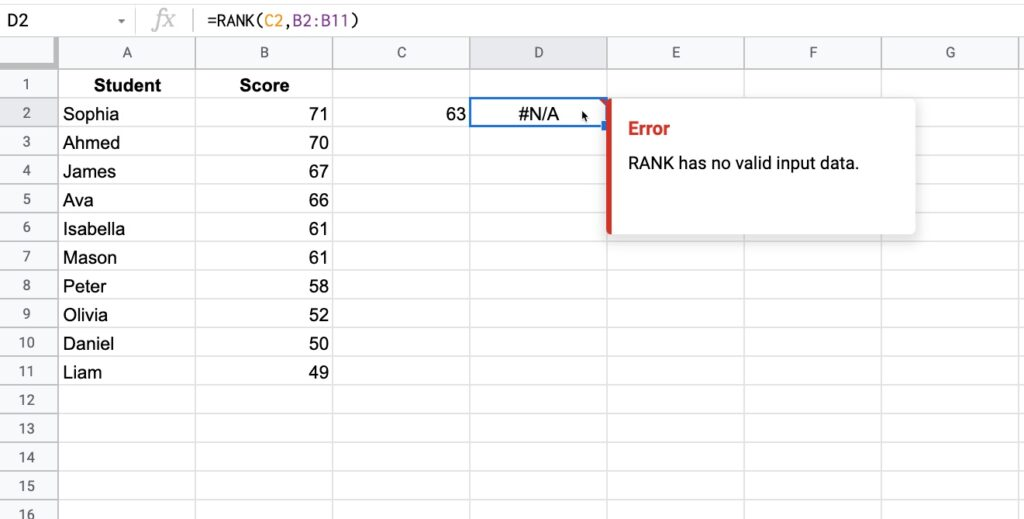Note: the sort order of the data does not affect the RANK.

## How To Use The RANK Function

Consider the following example, where the RANK function calculates the position of each student within the class: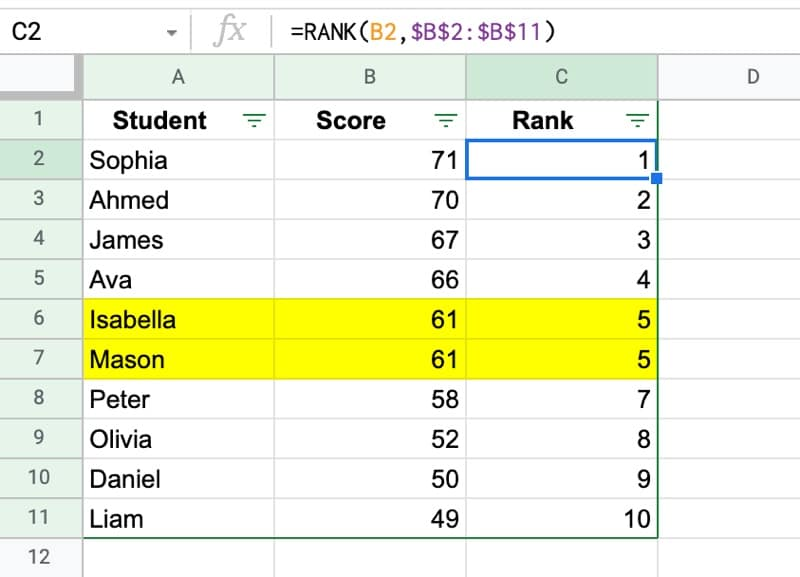Although the data is sorted from high to low scores, this is not required for the RANK function to work.

The RANK formula used in this example:

`=RANK(B2,\$B\$2:\$B\$11)`

Note that the third argument has been omitted, which means the largest value in the dataset is ranked 1.

To switch the ranking so that the smallest value is ranked 1, set the third argument in the RANK function to 1 or TRUE, e.g.:

`=RANK(B2,\$B\$2:\$B\$11,1)`

## RANK.EQ Function Example

With the same dataset, the RANK.EQ function gives the same output as the RANK function above: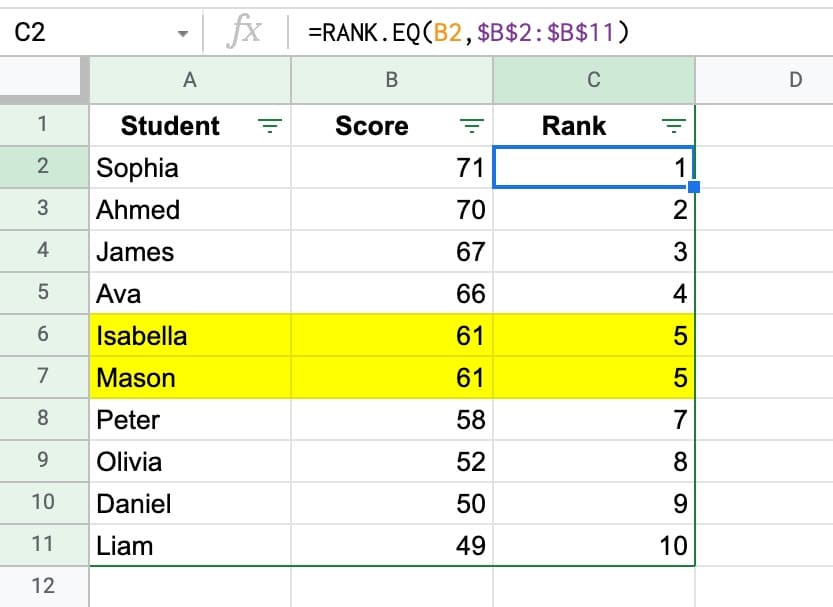Here, the RANK.EQ formula is:

`=RANK.EQ(B2,\$B\$2:\$B\$11)`

## RANK.AVG Function Example

Again, using the same dataset, here is the RANK.AVG function, which shows an average tied position: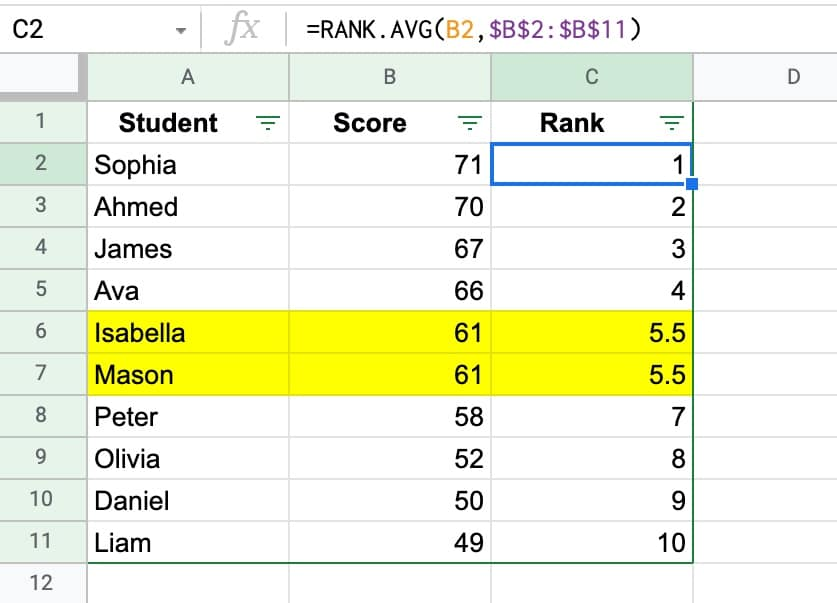In this case the RANK.AVG formula is:

`=RANK.AVG(B2,\$B\$2:\$B\$11)`

## RANK Formula with Arrays

In addition to ranges of data, the RANK functions accept arrays built using array literals `{...}`

For example, consider the following example where the same data is used, but inside an array:

`=RANK.EQ(67,{61,50,70,71,49,52,66,67,61,58})`

## RANK Function Template

Feel free to make a copy: File > Make a copy…

If you can’t access the template, it might be because of your organization’s Google Workspace settings. Right-click the link to open it in an Incognito window to see it.

The RANK function is also covered in the Day 26 lesson of my free Advanced Formulas 30 Day Challenge course.

The RANK, RANK.EQ, and RANK.AVG functions are part of the Statistical family of functions in Google Sheets.

1.carlos says:
1.Vlad says: